Ch 5. Rigid Body General Motion Multimedia Engineering Dynamics Fixed Axis Rotation Plane Motion Velocities Zero Velocity Point Plane Motion Accelerations Multiple Gears Rot. Coord. Velocities Rot. Coord. Acceleration
 Chapter - Particle - 1. General Motion 2. Force & Accel. 3. Energy 4. Momentum - Rigid Body - 5. General Motion 6. Force & Accel. 7. Energy 8. Momentum 9. 3-D Motion 10. Vibrations Appendix Basic Math Units Basic Equations Sections Search eBooks Dynamics Fluids Math Mechanics Statics Thermodynamics Author(s): Kurt Gramoll ©Kurt GramollDYNAMICS - CASE STUDY SOLUTION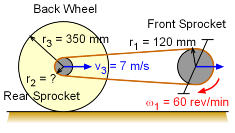Problem Diagram First, break the problem into three parts that can be related to one another. Front Sprocket, r1 Rear Sprocket, r2 Back Wheel, r3 Next analyze each part and relate their tangent and angular velocities. The angular velocity for the back sprocket and back wheel must be equal (assuming no slipping) and the tangent velocity of the two sprockets must be equal.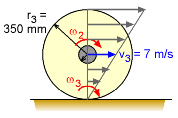Back Sprocket (disk 3)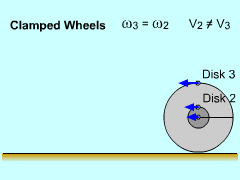Back Wheel Part a) Back Wheel Since the back wheel does not slip, the point of contact with the ground has zero velocity for that instant. The wheel is essentially rotating about the contact point, and the angular velocity ω3 can be calculated as      ω3 = v3/r3 = 7/0.350 = 20 rad/s It should be noted the center velocity v3 is relative to the ground. The angular velocity of the back wheel is the same as the velocity of the rear sprocket since they are fixed together.      ω2 = ω3                                                     (1) Part b) Front Sprocket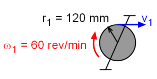Front Sprocket (disk 1)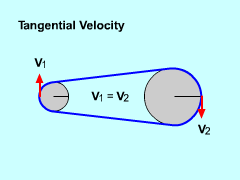Tangential Velocity of Both Disks are Equal The velocity of the outside edge of the front sprocket can be obtained by using the basic equation for rotation about a fixed axis.      v1 = ω1 r1 But first, the given angular velocity needs to be converted to standard units.      ω1 = 60 rev/min = 6.28 rad/s Substituting into the previous equation,      v1 = (6.28 rad/s)(120 mm) = 754 mm/s The velocity v1 is relative to the center of the sprocket, which is moving with the bike. This will not affect the final answer since the rear sprocket is also moving with the bike. Since the rear sprocket and front sprocket are connected by a chain, the tangential velocity of their edges will be the same      v1 = v2                                                        (2) Part c) Rear Sprocket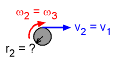Rear Sprocket (disk 2) Finally, the rear sprocket size can now be calculated since its angular velocity ω2 and tangential velocity v2 are known. The radius for the rear sprocket is      r2 = v2 / ω2         = (754 mm/s)/(20 rad/s)         = 37.7 mm It should be noted, that this exact size may not be available since the gear has teeth that come in increment sizes.

Practice Homework and Test problems now available in the 'Eng Dynamics' mobile app
Includes over 400 problems with complete detailed solutions.
Available now at the Google Play Store and Apple App Store.